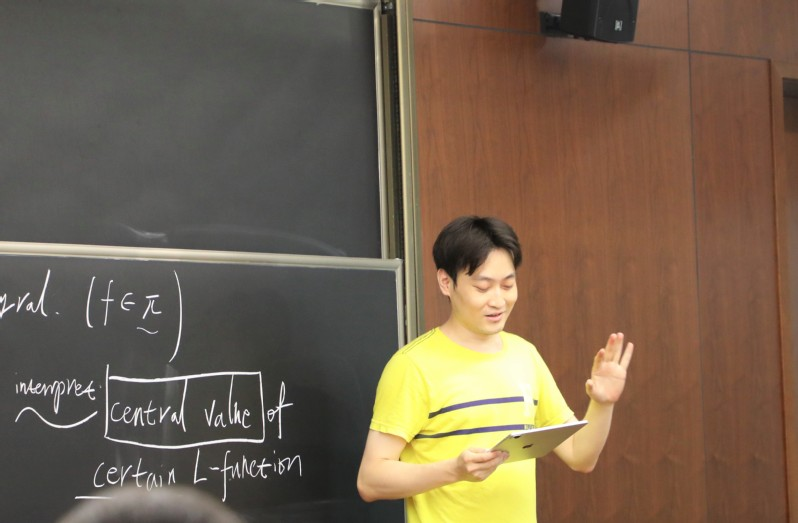Linear Arithmetic Fundamental Lemma and Intersection Numbers for CM Cycles on Lubin-Tate Spaces

BYThe central values and derivatives of L-functions are studied in the global Gan-Gross-Prasad conjecture and its arithmetic generalizations. These type of problems originally started from the study of Waldspurger furmula and Gross-Zagier formula. Recently, in the function field case, Yun and Zhang have discovered a higher Gross--Zagier formula, relating higher derivatives of L-functions to intersection numbers of special cycles on the moduli space of Drinfeld Shtukas of rank two.

The Guo-Jacquet Fundamental Lemma is a higher dimensional generalization of the local field analogue of the Waldspurger formula. It has an arithmetic generalization called the Linear Arithmetic Fundamental Lemma. We will introduce the known results for the linear Arithmetic Fundamental Lemma, and the intersection number formula for Lubin-Tate spaces. After a joint work with Ben Howard, we also discovered a bi-quadratic generalization of the conjecture.